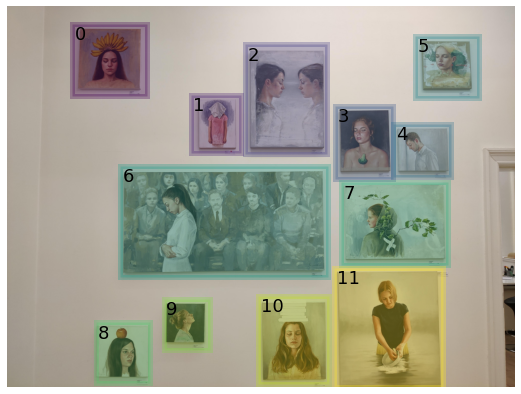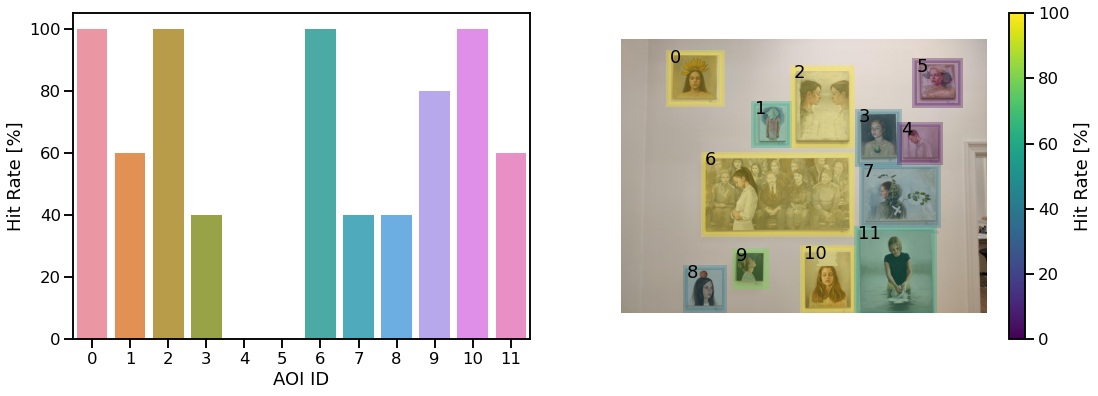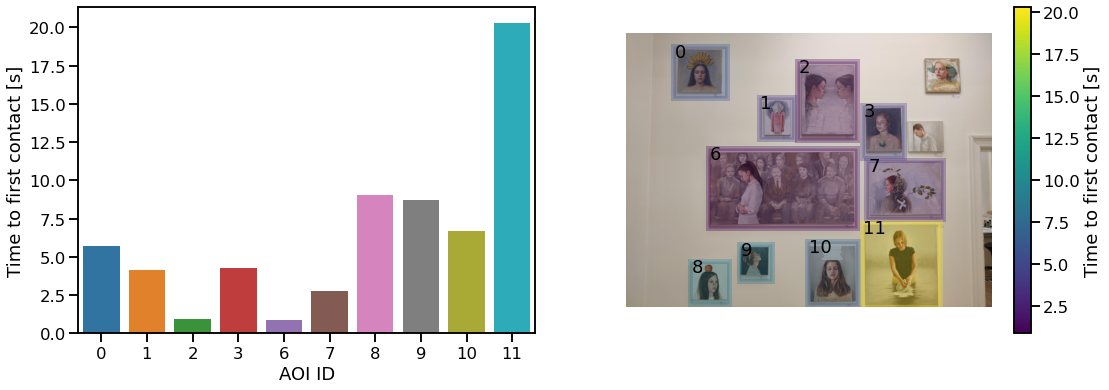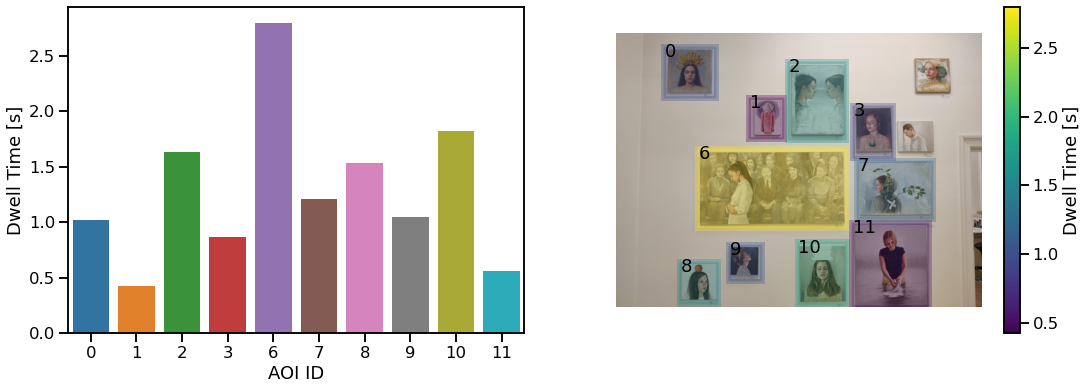# # Define AOIs and Calculate Gaze Metrics

Products used:

After mapping gaze to the environment, e.g. by using the Reference Image Mapper, it is a common goal to compare gaze behavior on different objects of interest within the environment. An easy way to facilitate this using the Reference Image Mapper is to define areas of interest (AOIs), also known as regions of interest (ROIs), in the reference image, and to compare them with various gaze metrics like e.g. dwell time.

In this guide, we will show you how to mark AOIs on a reference image, how to label which AOI a fixation was made on, and how to aggregate metrics from fixation data inside these AOIs.

As an example we will use the recordings and enrichment of the art gallery study available in the demo workspace. We will address questions like "How many visitors looked at each painting?", "How soon after arriving did visitors look at each painting?", and "How long did visitors look at each painting?".

## # Dependencies of this Guide

You can find all code from this guide as well as the used example data here. The example data originates from the Reference Image Mapper enrichment called `multiple_paintings_standing` located in the demo workspace here.

To execute it you need to have the following libraries installed:

`pip install matplotlib pandas opencv-python seaborn`

## # Define AOIs in the Reference Image

The reference image `reference_image.jpeg` is located inside the enrichment folder.

An easy way to define AOIs in the image is to use the `cv2.selectROIs` method from OpenCV. When executing this method, a window with the reference image will appear. Mark the AOIs by dragging rectangles over the areas you want your AOIs to cover. Hit the space key after each AOI and once you are done close the selection with the escape key.

``````import cv2
reference_image = cv2.cvtColor(reference_image_bgr, cv2.COLOR_BGR2RGB)
# resize the image before labelling AOIs makes the image stay in the screen boundaries
scaling_factor = 0.25
scaled_image = reference_image_bgr.copy()
scaled_image = cv2.resize(
scaled_image, dsize=None, fx=scaling_factor, fy=scaling_factor
)
# mark the AOIs
scaled_aois = cv2.selectROIs("AOI Annotation", scaled_image)
cv2.destroyAllWindows()
# scale back the position of AOIs
aois = scaled_aois / scaling_factor
print(scaled_aois)
``````

If you want to use the same AOIs as this guide is using, you can uncomment the following code rather than defining your own AOIs.

``````import numpy as np
# aois = np.array(
#     [[ 512.,  136.,  588.,  572.],
#     [1448.,  700.,  392.,  456.],
#     [1872.,  300.,  652.,  864.],
#     [2584.,  788.,  456.,  576.],
#     [3044.,  928.,  452.,  416.],
#     [3212.,  232.,  504.,  492.],
#     [ 892., 1256., 1636.,  880.],
#     [2628., 1388.,  844.,  648.],
#     [ 696., 2492.,  428.,  476.],
#     [1232., 2304.,  364.,  408.],
#     [1980., 2280.,  556.,  700.],
#     [2576., 2064.,  856.,  936.]]
# )
``````

## # Overlaying Areas of Interest on the Reference Image

Now, we visually check whether the AOIs we defined match up with our reference image. We define a `plot_color_patches` function, which we will use throughout the rest of this guide.

``````import matplotlib as mpl
import matplotlib.pyplot as plt
from matplotlib import patches
import seaborn as sns
sns.set_context("talk")
import pandas as pd
import numpy as np
def plot_color_patches(
image,
aoi_positions,
aoi_values,
ax,
alpha=0.3,
colorbar=False,
unit_label=""
):
ax.imshow(image)
ax.axis("off")
# normalize patch values
aoi_values_normed = aoi_values.astype(np.float32)
aoi_values_normed -= aoi_values_normed.min()
aoi_values_normed /= aoi_values_normed.max()
colors = mpl.cm.get_cmap("viridis")
# for patch_idx, (aoi, value) in enumerate(zip(patch_position, patch_values_normed)):
for aoi_id, aoi_val in aoi_values_normed.iteritems():
aoi = aoi_positions[aoi_id]
patches.Rectangle(
aoi,
*aoi[2:],
alpha=alpha,
facecolor=colors(aoi_val),
edgecolor=colors(aoi_val),
linewidth=5
)
)
ax.text(aoi + 20, aoi + 120, f"{aoi_id}", color="black")
if colorbar:
norm = mpl.colors.Normalize(vmin=aoi_values.min(), vmax=aoi_values.max())
cb = plt.colorbar(mpl.cm.ScalarMappable(norm=norm, cmap=colors), ax=ax)
cb.set_label(unit_label)
``````
``````plt.figure(figsize=(10, 7))
aoi_ids = pd.Series(np.arange(len(aois)))
plot_color_patches(reference_image, aois, aoi_ids, plt.gca())
``````## # Map Fixations to AOIs

Next up, we want to match the fixation coordinates to the AOIs. This is comparable to asking for every x/y coordinate pair that defines a fixation if that pair is inside any of the rectangles.

``````import pandas as pd
from IPython.display import HTML
# filter for fixations that are in the reference image
fixations = fixations[fixations["fixation detected in reference image"]]
fixations["AOI"] = None
def check_in_rect(fixation_data, rectangle_coordinates):
rect_x, rect_y, rect_width, rect_height = rectangle_coordinates
x_hit = fixation_data["fixation x [px]"].between(rect_x, rect_x + rect_width)
y_hit = fixation_data["fixation y [px]"].between(rect_y, rect_y + rect_height)
return x_hit & y_hit
for aoi_id, aoi in enumerate(aois):
fixations.loc[check_in_rect(fixations, aoi), "AOI"] = aoi_id
HTML("<div class='grid'>" + html + "</div>")
``````
section id recording id fixation id start timestamp [ns] end timestamp [ns] duration [ms] fixation detected in reference image fixation x [px] fixation y [px] AOI
0 3cd54e6f-f12e-468c-9a7b-6afffd5f0379 54a0deee-23dd-48f4-806e-6fdb519d6a7c 248 1636035560036503914 1636035560236515914 200 True 2419.0 2138.0 None
1 3cd54e6f-f12e-468c-9a7b-6afffd5f0379 54a0deee-23dd-48f4-806e-6fdb519d6a7c 249 1636035560256651914 1636035560480505914 223 True 2224.0 1717.0 2
2 3cd54e6f-f12e-468c-9a7b-6afffd5f0379 54a0deee-23dd-48f4-806e-6fdb519d6a7c 250 1636035560500491914 1636035560740669914 240 True 2427.0 1559.0 2
3 3cd54e6f-f12e-468c-9a7b-6afffd5f0379 54a0deee-23dd-48f4-806e-6fdb519d6a7c 251 1636035560776494914 1636035560972502914 196 True 1935.0 1119.0 None
4 3cd54e6f-f12e-468c-9a7b-6afffd5f0379 54a0deee-23dd-48f4-806e-6fdb519d6a7c 252 1636035561008557914 1636035561168510914 159 True 2828.0 1668.0 None

You can now see the new column "AOI". This column indicates whether gaze fell inside a given AOI.

## # Aggregating Fixation Metrics Inside AOIs

Using the defined AOIs and the mapped fixation data we can now calculate various metrics to compare gaze behaviour on the AOIs.

### # AOI Hit Rate

The Hit Rate of an AOI is defined as the proportion of subjects that looked at the AOI at least once. A Hit Rate of 100% means, every subject we recorded looked at the AOI, a Hit Rate of 50% indicates that only every second subject looked at the AOI, etc.

``````hits = fixations.groupby(["recording id", "AOI"]).size() > 0
hit_rate = hits.groupby("AOI").sum() / fixations["recording id"].nunique() * 100
# AOIs that have never been gazed at do not show up in the fixations data
# so we need to set them to 0 manually
for aoi_id in range(len(aois)):
if not aoi_id in hit_rate.index:
hit_rate.loc[aoi_id] = 0
hit_rate.sort_index(inplace=True)
``````
``````AOI
0    100.0
1     60.0
2    100.0
3     40.0
4      0.0
dtype: float64
``````
``````fig, ax = plt.subplots(ncols=2, figsize=(18, 6))
sns.barplot(x=hit_rate.index, y=hit_rate, ax=ax)
ax.set_xlabel("AOI ID")
ax.set_ylabel("Hit Rate [%]");
plot_color_patches(reference_image, aois, hit_rate, ax, colorbar=True, unit_label="Hit Rate [%]")
``````### # Time to First Contact

Time to First Contact measures how long it took observers to look at an AOI for the first time. Short times to first contact mean that the observers looked at the AOI early during the section.

We can compute time to first contact as the difference between the time when the first fixation was registered on an AOI and the time when the section started. So first, we need the information when each section started.

``````sections = pd.read_csv("data/sections.csv")
sections.set_index("section id", inplace=True)
# compute the difference for the respective section
for section_id, start_time in sections["section start time [ns]"].iteritems():
fixation_indices = fixations[fixations["section id"] == section_id].index
fixations.loc[fixation_indices, "aligned timestamp [s]"] = (fixations.loc[fixation_indices, "start timestamp [ns]"] - start_time) / 1e9
``````

Next, we aggregate the fixation data by section id and AOI, and extract the lowest value from the "aligned timestamp [s]" column. This is the time when the very first fixation on that AOI appeared in this session.

``````first_contact = fixations.groupby(["section id", "AOI"])["aligned timestamp [s]"].min()
first_contact = first_contact.groupby("AOI").mean()
first_contact
``````
``````AOI
0      5.717699
1      4.106685
2      0.924827
3      4.236960
6      0.880846
7      2.774175
8      9.038934
9      8.679888
10     6.706401
11    20.301783
Name: aligned timestamp [s], dtype: float64
``````
``````fig, ax = plt.subplots(ncols=2, figsize=(18, 6))
sns.barplot(x=first_contact.index, y=first_contact, ax=ax)
ax.set_xlabel("AOI ID")
ax.set_ylabel("Time to first contact [s]");
plot_color_patches(reference_image, aois, first_contact, ax, colorbar=True, unit_label="Time to first contact [s]")
``````### # Dwell Time

Dwell Time describes the total time an observer has looked at a given AOI on average. We can find the dwell time for each subject by summing up the durations of all fixations we detected, separately for each AOI and subject. Then we calculate the mean over all subjects.

``````dwell_time = fixations.groupby(["recording id", "AOI"])["duration [ms]"].sum()
dwell_time = dwell_time.groupby("AOI").mean()
dwell_time /= 1000
``````
``````AOI
0    1.0240
1    0.4230
2    1.6328
3    0.8660
6    2.7958
Name: duration [ms], dtype: float64
``````
``````fig, ax = plt.subplots(ncols=2, figsize=(18, 6))
sns.barplot(x=dwell_time.index, y=dwell_time, ax=ax)
ax.set_xlabel("AOI ID")
ax.set_ylabel("Dwell Time [s]");
plot_color_patches(reference_image, aois, dwell_time, ax, colorbar=True, unit_label="Dwell Time [s]")
``````